# 基于Qt的小目标探测声纳显控软件设计Display and Control Software Design of Small Target Detection Sonar Based on Qt

DOI: 10.12677/SEA.2020.96060, PDF, HTML, XML, 下载: 97  浏览: 513

Abstract: Sonar display and control software is an important part of sonar system. This paper introduces the design and implementation of the small target detection sonar display and control software based on Qt. The software architecture, data transmission, image display and processing methods are described, and static target suppression method is used to effectively reduce the false alarm rate, which can better meet the needs of users. It provides an effective reference for subsequent display control system of small target detection sonar.

1. 引言

2. 基于Qt的显控软件构架

Qt是一种面向对象、先进的、跨平台图形库软件 ，具有广阔的应用前景。Qt支持VxWorks、Windows、Linux等操作系统，只需通过交叉编译后就可使源代码在不同平台上运行。而且Qt模块化程度高并同时支持OpenGL、OpenCV等 ，对于开发人员而言十分便捷且友好，因此选择Qt作为本文显控软件的开发平台。Figure 1. Display and control software architecture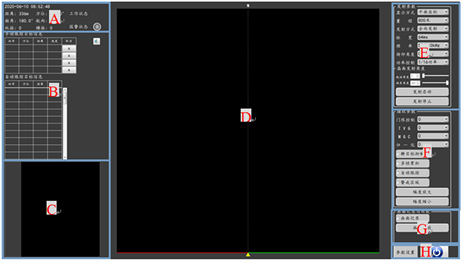Figure 2. Display and control software interface

A信息显示区：包含当前时间、光标位置信息、航速、航向、纵横摇信息、工作状态及报警状态显示。

B跟踪目标信息显示区：显示当前手动或自动跟踪目标的批号、距离、方位、速度信息。

C放大区：以光标为中心将回波放大显示。

D回波显示区：显示回波信息数据。

E发射参数设置区：包含显示方式、量程、发射方式、脉宽、频率、俯仰角度、功率控制、扇面发射角度设置、发射启动、发射停止控制。

F接收参数设置区：包含门限、TVG、MGC、归一化、静目标抑制、多帧累积、自动跟踪、警戒区域设置、幅度放大、幅度缩小控制。

G画面记录及回放区：包含画面记录及画面回放显示。

F参数设置及关机区：参数设置及关机按钮。

2.1. 参数显示

Qlabel是Qt中最基本的小部件类。一般用于显示当前日期时间、光标位置信息等标签文本，Qt默认坐标系原点位于左上角。X轴从左向右增长，Y轴从上到下增长。通过mousemoveevent事件，获得光标当前位置的坐标信息。

QTablwidget是一种常见的Qt程序显示数据表空间，类似于vc，c#中的datagrid，通常用于大容量文本跟踪目标信息等。实现跟踪目标信息实时更新显示。

2.2. 画面显示

{

tempPix = pix;

QPainter pp(&tempPix);

pp.drawRect(x,y,w,h);

painter.drawPixmap(0,0,tempPix);

}

else

{

QPainter pp(&pix);

pp.drawRect(x,y,w,h);

painter.drawPixmap(0,0,pix);

}

2.3. 控制菜单

QComboBox提供了一种以占用最少屏幕空间的方式向用户显示选项列表的方法。组合框是一个显示当前项目的选择小部件，可以弹出可选项目列表。组合框可以是可编辑的，允许用户修改列表中的每个项目。采用currentIndexChanged(int)及activated(int)实现量程、频率、发射方式等复数选择框。

QPushButton控件是一个非常常用的按钮控件，它继承自QABstractButton。采用clicked()实现发射控制、幅度控制。

3. UDP数据传输

Receiver= new QUDPSocket(this)；

Recevier->bind();//设置端口号

int i=30*1024*1024；

::setsockopt(receiver->socketDescriptor(),SOL_

SOCKET,SO_RCVBUF,(char*)&i,sizeof(i));//

Connection(reciver,SINGNAL(readyRead()),this,SLOT(processPendingDatagram);

void MainWindow::processPendingDatagram()

{

QByteArray datagram;

Datagram.resize(receiver->pendingDatagramSize();

If((datagram==//数据头及标志位)

{

Pdatal=datagram[];//波束批次数据低位

Pdata2=datagram[];//波束批次数据高位

a2=Pdata1+Pdata2*256；

for(a1=0；a1

{

H[a1][a2]= datagram[]//数据低位+ datagram[]//数据高位

}

4. 嵌入海图

glTexImage2D(GL_TEXTURE_2D,//指定纹理目标

0, //指定多级渐远纹理的级别

GL_RGB, //纹理储存为RGB值

width, height, //图像高度及宽度

0,

GL_RGB,//源图格式

GL_UNSIGNED_BYTE, //源图数据类型

data);//源图数据

glGenerateMipmap(GL_TEXTURE_2D);

5. 静态目标抑制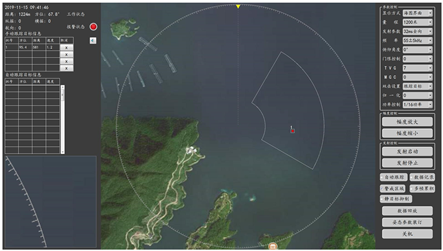Figure 3. Chart interface display

$P\left({X}_{t}\right)=\underset{i=1}{\overset{K}{\sum }}{\omega }_{i,t}\ast \eta \left({X}_{t},\text{\hspace{0.17em}}{\mu }_{i,t},\text{\hspace{0.17em}}{\sum }_{i,t}\right)$

$\text{\hspace{0.17em}}\eta \left({X}_{t},{\mu }_{i,t},{\sum }_{i,t}\right)=\frac{1}{{\left(2\pi \right)}^{\frac{n}{2}}{|\sum |}^{\frac{1}{2}}}{\text{e}}^{-\frac{1}{2}{\left(X-\mu \right)}^{T}{\sum }^{-1}\left(X-\mu \right)}$

${\omega }_{k,t}=\left(1-\alpha \right){\omega }_{k,t-1}+\alpha \left({M}_{k,t}\right)$

$\rho =\alpha \ast \eta \left({X}_{t}|{\mu }_{k},\text{\hspace{0.17em}}{\sigma }_{k}\right)$

${\mu }_{t}=\left(1-\rho \right)\ast {\mu }_{t-1}+\rho \ast {X}_{t}$

${\sigma }_{t}^{2}=\left(1-\rho \right)\ast {\sigma }_{t-1}^{2}+\rho \ast {\left({X}_{t}-{\mu }_{t}\right)}^{T}\left({X}_{t}-{\mu }_{t}\right)$

$B=\mathrm{arg}\left(\mathrm{min}\left(\underset{k=1}{\overset{b}{\sum }}{w}_{k}\succ T\right)\right)$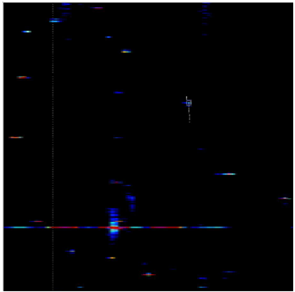Figure 4. Original image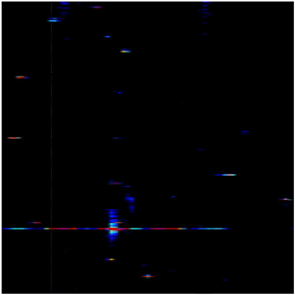Figure 5. Gaussian mixture background model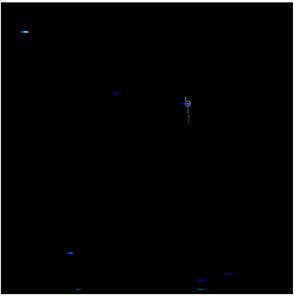Figure 6. Image after static target suppression

6. 结束语

NOTES

*通讯作者。

  刘娜, 肖雅静. 三维成像声纳显控软件系统设计[J]. 电子工业专用设备, 2017, 46(2): 52-54.  邓子强, 王玉玫, 邓红艳, 张宇. 基于Qt绘图系统的图形应用优化研究与实现[J]. 软件, 2016, 37(12): 59-62.  孙裕超, 张学磊, 田甜, 王文琮. 多波束成像声呐显控软件设计与实现[J]. 电声技术, 2017,41(3): 82-87.  冯贝. 基于Qt的分布式雷达生命探测显控软件设计[D]: [硕士学位论文]. 成都: 成都理工大学, 2019.  陈艳静. 探鱼声纳系统综合显控软件[D]: [硕士学位论文]. 哈尔滨: 哈尔滨工程大学, 2014.  范超男, 李士心, 张海, 等. 基于改进的混合高斯模型运动目标检测算法研究[J]. 天津职业技术师范大学学报, 2019(4): 25-31.